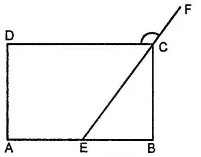The measures of two adjacent angles of a parallelogram are in the ratio 3:2. Find the measure of each of the angles of the parallelogram.

Asked by Aaryan | 1 year ago |  80

##### Solution :-

Let the measures of two adjacent angles, ∠A and ∠B, of parallelogram ABCD are in the ratio of 3:2. Let ∠A = 3x and ∠B = 2x

We know that the sum of the measures of adjacent angles is 180 º for a parallelogram.

∠A + ∠B = 180º

3x + 2x = 180º

5x = 180º

x = $$\frac{180^\circ}{5} = 36^\circ$$

∠A = ∠C = 3x = 108º (Opposite angles)

∠B = ∠D = 2x = 72º (Opposite angles)

Thus, the measures of the angles of the parallelogram are 108º, 72º, 108º, and 72º

Answered by Sakshi | 1 year ago

### Related Questions

#### In the given figure, PQRS is a kite. Find the values of x and y.

In the given figure, PQRS is a kite. Find the values of x and y.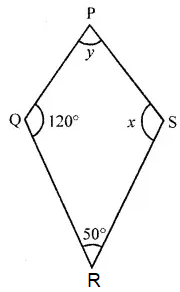#### In the given isosceles trapezium ABCD, ∠C = 102°. Find all the remaining angles of the trapezium.

In the given isosceles trapezium ABCD, ∠C = 102°. Find all the remaining angles of the trapezium.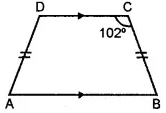#### In the given figure, ABCD is a rhombus and ∠ABD = 50°. Find :

In the given figure, ABCD is a rhombus and ∠ABD = 50°. Find :

(i) ∠CAB

(ii) ∠BCD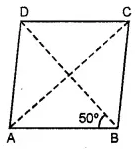#### In the given figure, ABCD is a rectangle and diagonals intersect at O. If ∠AOB = 118°, find

In the given figure, ABCD is a rectangle and diagonals intersect at O. If ∠AOB = 118°, find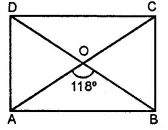(i) ∠ABO

(iii) ∠OCB